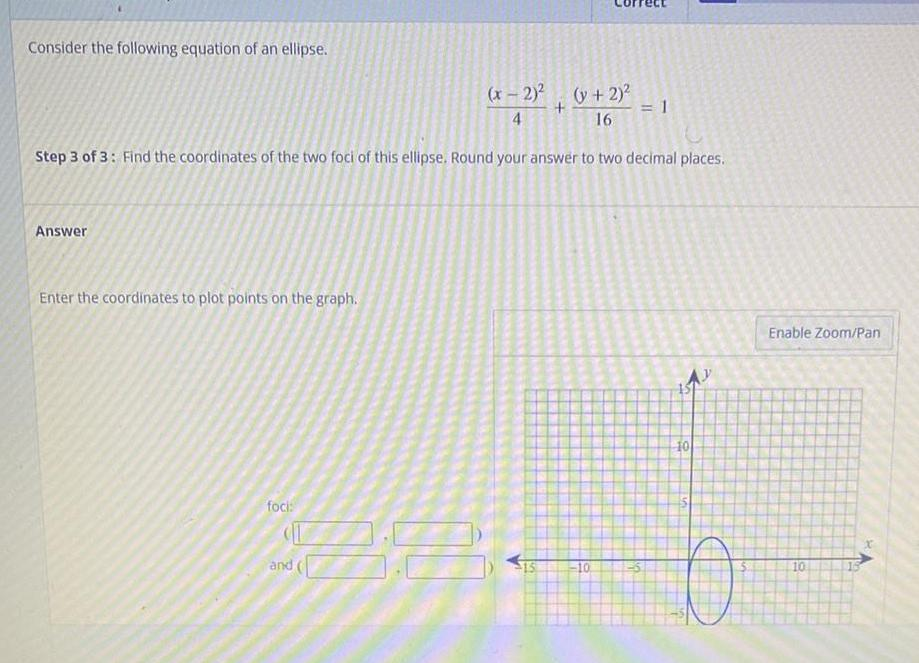Question:

# Consider the following equation of an ellipse. (x - 2)²/4+(y

Last updated: 8/12/2022Consider the following equation of an ellipse. (x - 2)²/4+(y + 2)² /16 =1 Step 3 of 3: Find the coordinates of the two foci of this ellipse. Round your answer to two decimal places Enter the coordinates to plot points on the graph.